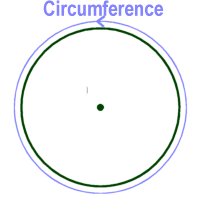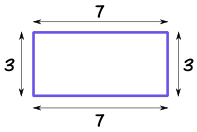# Mathematics Scheme of Work, SS1, 3rd Term

### Scheme of Work (Mathematics) SS1, 3rd Term:

#### WEEK 1

Topic: Deductive Proofs

• Types and properties of triangles
• Proofs of sum of angles in a triangle is 180o, the exterior angles is equal to the sum of its two interior opposite angles.

#### WEEK 2

Topic: Deductive Proofs

• Similar and congruent triangles
• Isosceles and equilateral triangles.

#### WEEK 3

Topic: Deductive Proofs

• Properties of parallelogram and related quadrilaterals.
• Intercept theorem
• Parallelogram of the same base and between the same parallel lines are equal in area.

#### WEEK 4

Topic: Polygon - (Types)

• Sum of interior angles of any n-sided polygon.
• Sum of exterior angles of any polygon
• Problem solving on polygon.

#### WEEK 5

Topic: Trigonometry

•  Basic trigonometric ratios, sine, cosine and tangent with respect to right-angled triangles.
• Trigonometric ratio of special angles 30o, 45o, 60o.
• Deriving trigonometric ratios of 30o, 45o, 60o

#### WEEK 6

Topic: Trigonometry

• Solving problems involving use of sine, cosine and tangent at right-angled triangles.
• Application of trigonometric ratios of 45o, 30o and 60o to solving problem without the use of calculating aids.

#### WEEK 7

Topic: Trigonometry

• Trigonometric ratios related to the unit circle
• Draw graphs of sine from  0o ≤ ө ≤ 360o
• Draw graphs of cosine from 0 ≤ ө ≤ 360o

#### WEEK 8

Topic: Statistics

• Revision on collection, tabulation and presentation of data.
• Construction of frequency tables
• Bar charts and histogram differentiate between bar chat and histogram.

#### WEEK 9

Topic: Statistics

• Calculating the sectoral component of pie chart.
• Drawing pie chart correctly.
• Interpreting the pie chart and bar chart.

#### WEEK 10

Topic: Statistics (Grouped Data)

• Drawing histogram
• Estimation of mode from histogram.

#### WEEK 11

Topic: Statistics

• Construction of frequency polygon of a given distribution.

• Revision

#### WEEK 13

• Examination

Mathematics scheme of work, SS1, 1st Term

Mathematics scheme of work, SS1, 2nd Term

Read topics on Mathematics here

THANKS FOR READING - Please Help Share!

#### NOTABLE POINTS IN Mathematics Circumference in mathematics means the distance around the edge of a circle (or a another curverd surface). Perimeter means the distance around a square or rectangle or polygon. Circumference is to circle or any other curved surfaceImage Credit: Ducksters Perimeter is to square or rectangle or polygonImage Credit: Maths is Fun

CONTRIBUTE TO THIS TOPIC | ASK A QUESTION